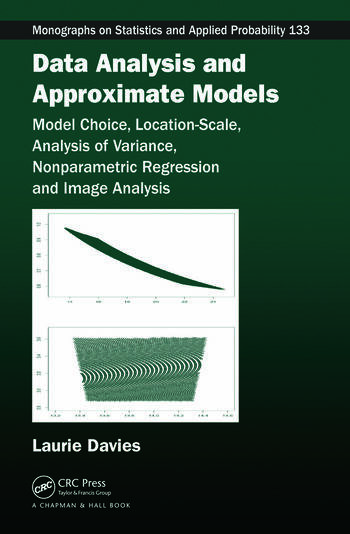# Data Analysis and Approximate Models: Model Choice, Location-Scale, Analysis of Variance, Nonparametric Regression and Image Analysis

## 1st Edition

Patrick Laurie Davies

Chapman and Hall/CRC
Published July 7, 2014
Reference - 320 Pages - 110 B/W Illustrations
ISBN 9781482215861 - CAT# K22027
Series: Chapman & Hall/CRC Monographs on Statistics and Applied Probability

USD\$115.00

FREE Standard Shipping!

## Preview

### Summary

The First Detailed Account of Statistical Analysis That Treats Models as Approximations

The idea of truth plays a role in both Bayesian and frequentist statistics. The Bayesian concept of coherence is based on the fact that two different models or parameter values cannot both be true. Frequentist statistics is formulated as the problem of estimating the "true but unknown" parameter value that generated the data.

Forgoing any concept of truth, Data Analysis and Approximate Models: Model Choice, Location-Scale, Analysis of Variance, Nonparametric Regression and Image Analysis presents statistical analysis/inference based on approximate models. Developed by the author, this approach consistently treats models as approximations to data, not to some underlying truth.

The author develops a concept of approximation for probability models with applications to:

• Discrete data
• Location scale
• Analysis of variance (ANOVA)
• Nonparametric regression, image analysis, and densities
• Time series
• Model choice

The book first highlights problems with concepts such as likelihood and efficiency and covers the definition of approximation and its consequences. A chapter on discrete data then presents the total variation metric as well as the Kullback–Leibler and chi-squared discrepancies as measures of fit. After focusing on outliers, the book discusses the location-scale problem, including approximation intervals, and gives a new treatment of higher-way ANOVA. The next several chapters describe novel procedures of nonparametric regression based on approximation. The final chapter assesses a range of statistical topics, from the likelihood principle to asymptotics and model choice.